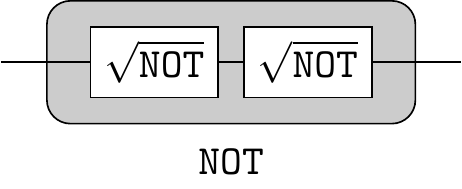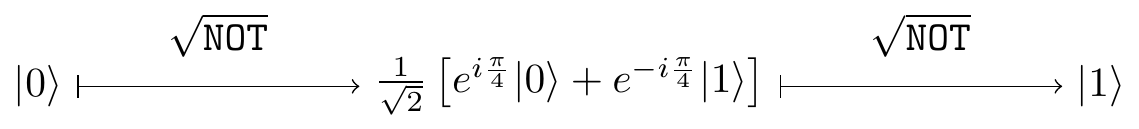## 2.8 From bit-flips to phase-flips, and back again

The Pauli Z gate is a special case of a phase gate P_\varphi with \varphi=\pi. When we insert it into the interference circuit we obtainIf you wish to verify this, write the Hadamard gate as H = (X+Z)/\sqrt{2} and use the properties of the Pauli operators. So the Hadamard gate turns phase-flips into bit-flips, but it also turns bit-flips into phase-flips:Let us also add, for completeness, that HYH=-Y. You will see these identities again and again, especially when we discuss quantum error corrections.56 \begin{aligned} HXH &= Z \\HZH &= X \\HYH &= -Y \end{aligned}

1. Unitaries, such as H, that take the three Pauli operators to the Pauli operators via conjugation form the so-called Clifford group, which we will meet later on, in Chapter 7. Which phase gate is in the Clifford group of a single qubit?↩︎